# Classification vs Regression

## Introduction

Supervised Machine learning algorithms are employed to tackle two kinds of tasks - Classification and Regression.

It’s fundamental for data scientists to clearly differentiate between classification and regression tasks to employ correct techniques to solve a certain problem. Regression problems have continuous output variables, that is, the outputs aren’t bound within a set of absolute values.

Suppose a model predicts house prices in Delhi in a specific month. Now, these house prices could take any value. It could be said that the output variable, in this case, is continuous, and hence this is a regression task.

On the other hand, classification tasks have categorical(or discrete) target variables. Like a model that predicts if a person would turn out to be a loan defaulter or not, based on which a bank can decide if they want to sanction their loan.

Both the tasks have specific algorithms which may or may not be suited for the other.

## Regression

As discussed earlier, regression tasks have continuous output variables. Regression analysis is often required in the domain of finance and investing in finding the relationship between independent variables and the dependent variable. Some of the popular regression algorithms are discussed below:-

### Linear Regression

Linear regression tries to find a linear relationship between the dependent and independent variables. The algorithm tries to find the best fit line for the data points on the X-Y plane. It is given by the formula:-

y=a0x+a1

Where,

y = dependent variable

x = independent variable

a= linear regression coefficient (slope)

a1 = y intercept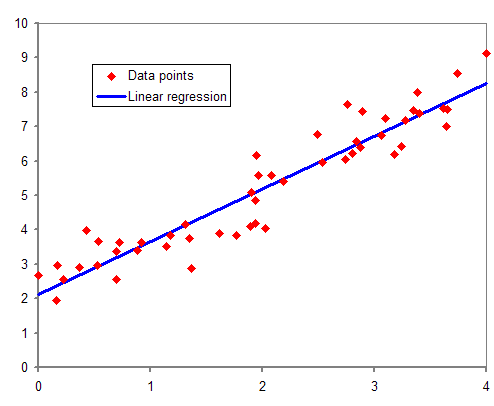Linear regression is of two types -

• Simple Linear regression:-  One independent and one dependent variable (x , y).
• Multiple linear regression:-  Several independent variables for one dependent variable ( (x0,x1,x2…..xn) , y).

To check how well the line depicts the relationship between the data points, a  cost function is used.

Mean Squared Error (MSE) = 1/N ∑(actual-forecast)2

N = total number of values

actual = actual value (as given in (x,y) coordinates)

forecast = predicted value (as predicted by the function a1.X + a)

The cost function checks for which values of ao and ais the function most accurate.

Pros

• Easy to implement.
• Less complex than other regression techniques.

Cons

• The accuracy of the algorithm is vulnerable to outliers.
• Real-world problems aren’t usually simple enough to form a linear relationship.

### Support Vector Regression(SVR)

You might have heard about Support Vector Machines(SVM). It’s a popular algorithm that is widely used for classification tasks. SVR uses a similar concept but to predict real values. The data points on the X-Y plane are segregated via hyperplanes. The dimensions can be increased in case this segregation is not possible.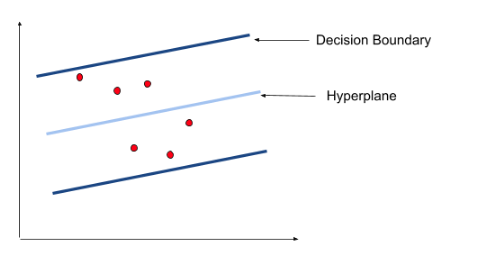Hyperplane is our best fit line with maximum data points. Consider Decision boundaries to be at a distance of ‘s’ from the hyperplane. Our primary task is to decide this distance so that points closest to the hyperplane are within the boundary lines. Hence we are going to consider only those points that are within the boundary line and have the least error.

Pros

• SVR can accommodate outliers.
• They have excellent generalisation capabilities.

Cons

• Not well suited for large datasets.
• Not well suited for datasets with a lot of noise.

### LASSO Regression

LASSO is an acronym for Least Absolute Selection Shrinkage Operator. Shrinkage here refers to shrinkage of parameters

LASSO applies constraints on attributes that cause regression coefficients of some variables to tend to zero.

Variables whose coefficients are shrunk to zero are neglected from the model. This basically means that these features are not important in deciding the best fit line of our model. So essentially it makes a feature selection.

Let us understand with an example.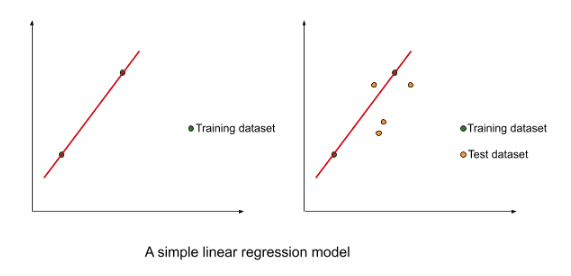In the above figure, we took a small dataset to train a simple linear regression model.

The best fit line had a very low bias. However, the results were underwhelming when the model was tested on the test dataset. There was a very high variance. This is the case of overfitting when the model has a low bias(the line fits training data very well) and high variance(the line doesn’t fit the testing data very well). This is owing to the cost function used in the simple linear regression.

Mean Squared Error (MSE) = 1/N ∑(actual-forecast)2

The cost function minimised the sum of residuals. But it leads to the case of overfitting.

LASSO regression tweaks that cost function a bit to tackle this problem of overfitting.

The cost function in LASSO regression is :-

Cost function = 1/N ∑(actual-forecast)2 +  λ |slope|

Where λ can take any value between 0 and infinity. The value is selected using cross-validation.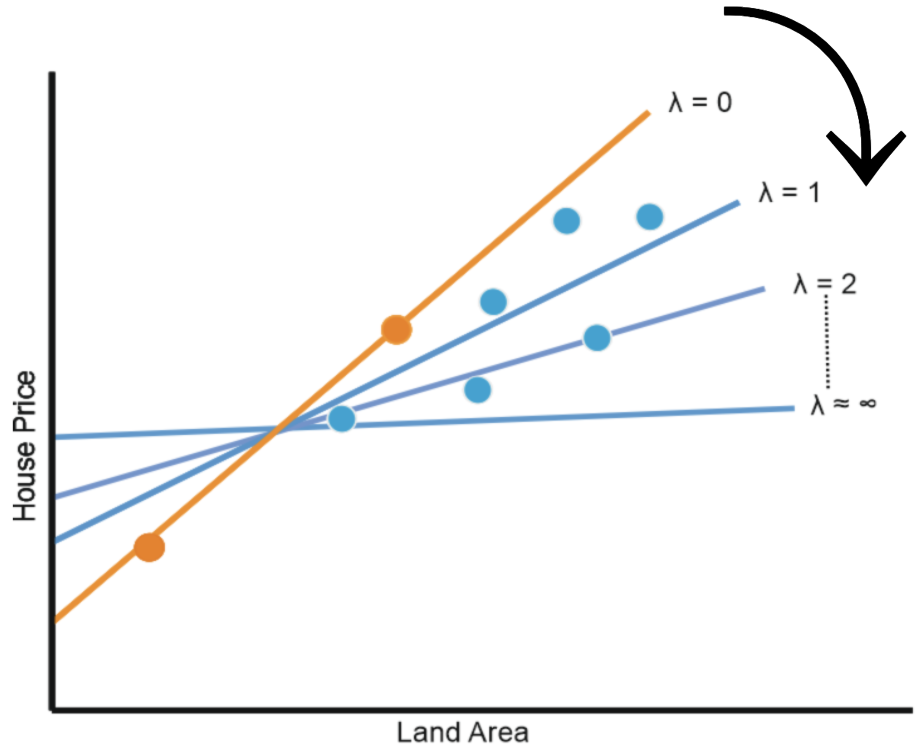The LASSO regression cost function penalises higher-order slopes. These higher-order slopes generally lead to overfitting. We need to keep in mind that our model needs to be a general model which makes uniform predictions throughout our training and testing data. So to reduce overfitting, we make a trade-off between bias and variance. In the case of overfitting, the bias was close to zero but variance was huge. The additional term in the cost function balances bias and variance, hence generalising our model.

Pros

• It helps overcome the problem of overfitting.

Cons

• Selected parameters can be highly biased.

### Ridge Regression

It is another regularisation technique, not much different from LASSO regression.

They differ just slightly in their cost function.

Cost function = 1/N ∑(actual-forecast)2 +  λ (slope)2

Ridge regression also addresses the problem of overfitting in linear regression by making the bias-variance tradeoff, Just like LASSO regression. And the value of λ can be between 0 to infinity and is chosen by cross-validation, just like LASSO regression.

However, they differ in shrinkage of the coefficients. In LASSO regression, the shrinkage of any coefficient could go all the way down to zero. But in Ridge regression, the shrinkage never goes all the way down to zero, no matter how high the value of the coefficient of penalty (λ) is.

So unlike LASSO regression where we were essentially doing feature selection by shrinking the values of some coefficients all the way down to zero, in ridge regression that is not the case. But it’s intuitive to learn that the smaller the value of the coefficient becomes, the lesser it will make in choosing in the final model.

## Classification

Classification tasks, as discussed earlier, have categorical or discrete-valued target variables. Most of the regression algorithms cannot be applied to classification tasks or vice versa. Even those that can be, aren’t very efficient with their predictions. Some of the classification techniques are discussed below:-

### Logistic Regression

It might sound counter-intuitive to think Logistic regression is actually a classification algorithm. That is, it is employed when the target variable is categorical. It gives the probabilistic values, i.e., its value lies between 0 and 1.  This is done with a logistic function. The function predicts the probability of an outcome. We decide a threshold value above which the outcome would be favourable and below which the outcome would be unfavourable.

Suppose an employer needs to check if a certain employee deserves a raise. The logistic function would generate a value between 0 and 1. We would decide a threshold value, say 0.5, above which the employee deserves a raise. And below which they don’t. Suppose the outcome of the algorithm is 0.7 (>0.5), the employee would get a raise. Now since there can be only two possible outcomes (raise or no raise), that makes this problem a classification problem.

Since all the values lie between 0 and 1(probabilistic function), it forms an S-like Curve called the sigmoid function.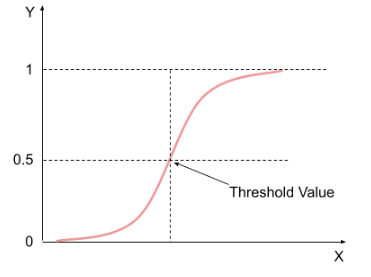It is given by

log[y/(1-y)] = b0 + b1x1 + b2x2 + b3x+ ……. + bnxn

There are various types of logistic regression such as binomial( 2 dependent variables), multinomial(3 or more unordered dependent variables), ordinal(3 or more ordered dependent variables).

### Decision Trees

A supervised learning algorithm that can be used for both, classification and regression tasks. But is mainly used for classification problems. Decision trees are tree-like classifiers where the internal nodes are decision nodes and the leaf nodes are outcome nodes.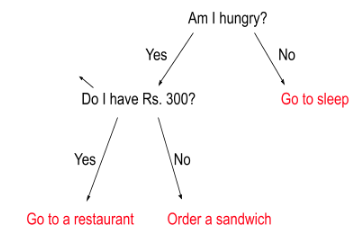In the above example, all the internal nodes are decision-makers while end or leaf nodes are outcomes. We can see how a decision tree comes to a conclusion. Now the question arises, how to decide which attribute should be at the root node and which attributes would become the further sub-nodes.

The more information a feature provides in making the prediction the better it is. So the feature which gives the maximum information is kept at the root level, and as we go down the tree the classification becomes even more granular. This process is known as Attribute Selection Measure (ASM). There are two popular ASM techniques:-

• Information gain-

Information gain is the measurement of changes in entropy after the segmentation of a dataset based on an attribute.

It calculates how much information a feature provides us about a class.

Higher the value of information gain for an attribute, the higher will be its priority. Or closer it would be to the root node.

Information Gain= Entropy(Total sample size)- [(Weighted Avg) *Entropy(each feature)]

Entropy is the measure of impurity in the data and can be calculated as

Entropy(s)= -P(yes)log2P(yes)- P(no) log2P(no)

• Gini Index:-

Another popular ASM technique which ranks the attributes based on their purity. Gini index calculates the impurity of an attribute and higher is the purity, the more relevant information the feature gives in making the prediction. Hence an attribute with a lower Gini index is given higher priority than an attribute with a higher Gini index.

It is given by the formula-

Gini Index= 1- ∑jPj2

Pros

• Their tree-like structure somewhat simulates a human thought process and hence makes them very intuitive.
• It can be used for both classification and regression tasks.
• Doesn’t require a very rigorous preprocessing of data.

Cons

• An optimal tree depth is an absolute necessity. Otherwise, it can lead to overfitting or underfitting.

### Random Forest Classifier

Random forest stems from the decision tree algorithm. It makes use of several different decision trees that generate their own prediction. These decision trees are formed using varying subsets of features from the main dataset. The mode of the predictions by all the decision trees is the final output of the random forest model.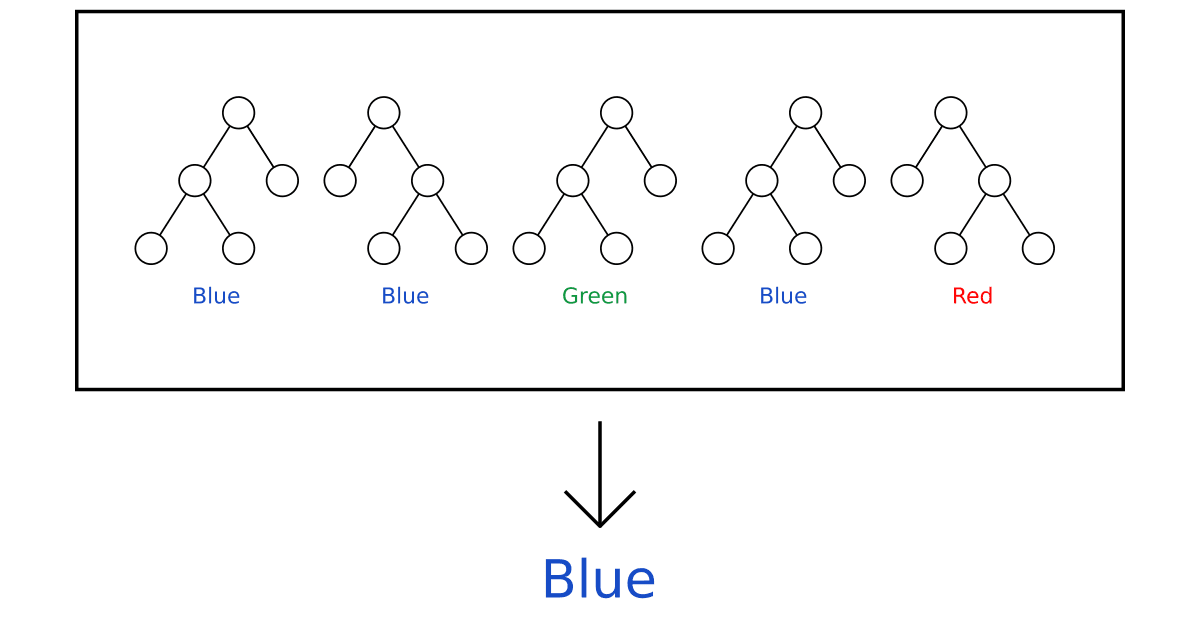Pros

• Very reliable with complex and huge datasets.
• Very Intuitive.
• Can be used for both classification and regression tasks.

Cons

• Computationally expensive.

### K Nearest Neighbours (KNN)

KNN is one of the simplest supervised machine learning algorithms that can be used for both classification and regression tasks. However, it is much more accurate for classification tasks.

KNN categorises the data points based on their distance from their k nearest neighbours.  It assumes the closer the data points are, the more similar they are to each other.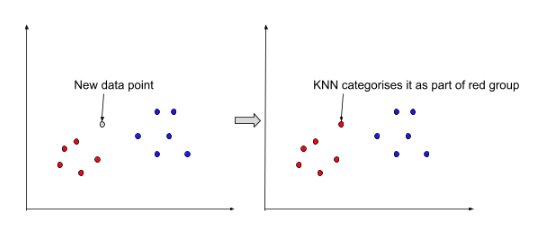The distance between the data points is the euclidean distance and the K value is decided iteratively for which the predictions are most accurate. We find the K neighbours of the data point and then count the number of data points in each category. The category from which maximum neighbours are from is assigned to the new data point.

Pros

• Easy to understand and implement.
• Can be used for both classification and regression tasks.

Cons

• Calculating the Euclidean distance of k neighbours can be computationally expensive.

Naive Bayes

It is a supervised learning algorithm used for classification tasks. It’s a probabilistic model because it makes use of the probability of an event to make predictions.

It gets its name from Bayes theorem using which it does all the computations. The Bayes theorem is given by the formula -

P(A|B) = P(B|A)P(A)

P(B)

Now let’s see how this is implemented in Naive Bayes algorithm. Suppose we have a dataset with n features with values {x1, x2, x3, ……,xn} for which there can be 2 possible outputs {y1, y2}.

We find the probabilities of all possible outputs given the values of the independent variables.

P(y1 | (x1 ,x,...,x3)) = [P(x1|y1)P(x2|y1)...P(xn|y1)] P(y1)                    ...(1)

P(x1)P(x2)...P(xn)

Similarly,

P(y2 | (x1 ,x,...,x3)) = [P(x1|y2)P(x2|y2)...P(xn|y2)] P(y2)                    …(2)

P(x1)P(x2)...P(xn)

Then

P(y1) = (1) / [(1) + (2)]

P(y2) = (2) / [(1) + (2)]

The greater of the two values ( between P(y1) and P(y2) ) is then chosen as our final prediction.

## Summary

1. What’s the primary difference between regression and classification?
Regression tasks have continuous output variables while classification tasks have discrete output variables.

2. Mention some of the algorithms for both kinds of tasks.
Regression:- Linear regression, LASSO regression, Ridge regression, etc.
Classification:- Decision tree, Random forest, KNN, Logistic regression, etc.

3. What is the difference between LASSO and Ridge regression?
LASSO regression - Cost function = 1/N ∑(actual-forecast)2 +  λ |slope|
Ridge Regression - Cost function = 1/N ∑(actual-forecast)2 +  λ (slope)2
Both Ridge and LASSO regression are regularisation techniques that overcome the problem of overfitting generally faced in simple linear regression algorithm. They both work on the same principle,  by making a bias and variance tradeoff by shrinking the coefficients or slopes. However, Ridge regression shrinkage can make the coefficients tend to zero but would never actually be zero no matter how big the value of the penalty coefficient is. In case of LASSO, it can go all the way down to zero.

## Key Takeaways

Supervised machine learning is a very vast domain in data science. It’s essential for data scientists to be able to clearly differentiate between classification and regression tasks. We did a detailed analysis of differences between both kinds of tasks and methodologies that can be employed in various scenarios.  We have covered some of the most popular classification and regression algorithms and discussed their advantages and their downsides as well. It’s essential to consider both, their pros and cons when choosing a suitable model.

Are you aspiring to build a career in one of the most in-demand technologies of the modern world? Check out our industry-oriented data science courses curated by industry experts to ace your next data science interview.

Happy Learning!!